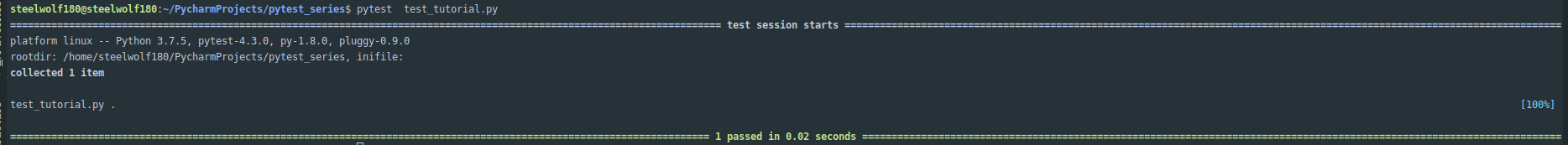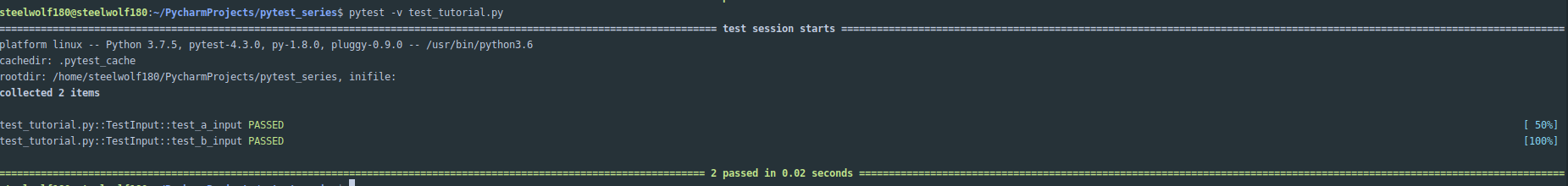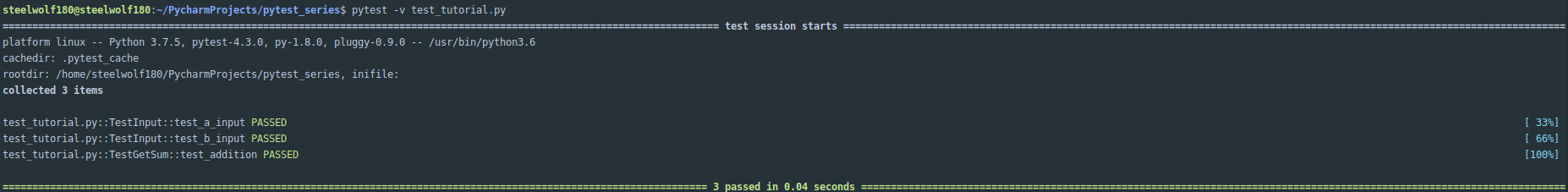# Introduction

Welcome back to part 2 of the test-driven development with PyTest.

For part two of the TDD with Pytest.

I would be covering the project structure where your test cases will reside.

The creation of test cases as a class or function under pytest.

Do head to part 1 of the series before proceeding with part 2.

It is assumed that a Linux system is used for this series.

Please use a Linux variant like Ubuntu or a cloud IDE like Codenvy running a Linux virtual container.

# Project Structure

Whenever you are creating test cases for your Python program.

There are the various school of thoughts on this for the location you will be putting your test cases in.

Your test cases could either reside within the program's subdirectories you are testing or create a centralized directory which your test cases reside in.

Some pros and cons come with it. It is a matter of project preference that is set by the development & QA team.

For me, I would prefer a centralized location for my test cases under ** tests** directory.

As it reduces the amount of time that a developer has to hunt and test out the various program components.

He did a combination of both for bite-sized consumption in each chapter and putting the test cases under specific test directory.

Therefore going through his book will allow you to consider which will be a better choice for you.

You could access the book's source code and use the book as a reference guide to help you learn about Pytest in Python.

# Create Test Cases as Class or Function

When you are just starting to learn to create test cases using Pytest.

We start by embedding a test case function to the program:

## Embedded Test Case as Function

Create a file called `tutorial.py` and with a `test_input` function:

``touch tutorial.py``

tutorial.py '''python a = 0 b = 0

def test_input(): assert a >= 0 assert b >= 0

''' Now run the following command in your own terminal.

``pytest tutorial.py``This is an example of creating your test cases as a function.

When a file has more than a certain amount of lines. It has to be separated for ease of maintainability.

## Test cases in a Separate File

Now refactor this test case into a separate file called `test_tutorial.py` which was created during part 1 of the series:

test_tutorial.py

``````import tutorial

def test_input():
a = tutorial.a
b = tutorial.b

assert a >= 0
assert b >= 0``````

Now execute the previous command.

``pytest test_tutorial.py``Besides importing the `tutorial.py` and adding variable declaration as part of the function.

## Test Cases in a Class

Classes are commonly used as a way to store multiple test cases under a specific feature or part of the program.

The reason for it is to allow testing of a specific part of the program.

Without another feature to interfere with your test cases that might lead to an inaccurate test result.

Now, let us refactor the previous function test case into a class function called `TestInput`:

``````import tutorial

class TestInput:
def test_a_input(self):
a = tutorial.a

assert type(a) != float
assert a >= 0

def test_b_input(self):
b = tutorial.b

assert type(b) != float
assert b >= 0``````

Now let us run the pytest command with the `-v (verbose)` option enabled on the newly refactored class test case.

``pytest -v test_tutorial.py``You should have two test cases which are `test_a_input` & `test_b_input` under `TestInput`.

Now for this `TestInput` class is used to test the input parameters of the program called `tutorial.py`.

We could create and test the function in the `tutorial.py` called `getsum` which calculates the result of parameter `a` and `b` as another class:

tutorial.py

``````a = 0
b = 0

def getsum(a, b):
return a + b``````

test_tutorial.py

``````import tutorial

class TestInput:
def test_a_input(self):
a = tutorial.a

assert type(a) != float
assert a >= 0

def test_b_input(self):
b = tutorial.b

assert type(b) != float
assert b >= 0

class TestGetSum:
assert tutorial.getsum(2, 8) == 10``````

Now let us run the following command, which results with 3 test cases under 2 classes.

``pytest -v test_tutorial.py``Imagine you could enhance it further by creating multiple test cases within each class. That is the reason why having a test case as a class is useful for maintainability purposes and separation of concern.

# Conclusion

I hope this article provides you with an understanding of how your test cases should reside for the project structure.

Along with the understanding of the difference creating test cases as a function or class for PyTest.

Do note that the next part of the series, I will show my attempt to use a PyTest for a code kata exercise to demonstrate your understanding of PyTest.